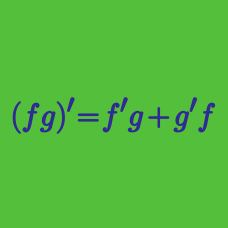Calculus

# Differentiation Rules: Level 3 Challenges

$f(x) = e^x \cdot \sin x$

For non-zero values of $f(x)$, simplify the expression below. $\log_{2} \dfrac{f^{(2016)} (x)}{f(x)}$

Notation: $f^{(n)}(x)$ denotes the $n^\text{th}$ derivative of $f(x)$.

$\large { \left. \frac { d }{ d(\cos { (x) } ) } \cos { (2015x) } \right\vert _{ x=2\pi } } = \ ?$

Let $f:\mathbb{R}\rightarrow \mathbb{R}$ be defined by $f(x) = x^3+3x+1$ and $g$ be the inverse of $f$. If the value of $g''(5)$ is equal to $\dfrac{-a}{b}$, where $a$ and $b$ are coprime positive integers, find the value of $a+b$.

Find a closed-form expression for the following derivative.

$\frac{d}{d(x^{n})}x^{y}$

${F(x)} = {f(x)g(x)h(x)}$

The above equation is true for all real $x$, where $f(x)$, $g(x)$ and $h(x)$ are differentiable functions at some point $a$.

Given $F '(a) = 21 F(a), f '(a) = 4f(a), g'(a) = -7g(a), h'(a) = kh(a)$. Find $k$.

×